Plotting the Linear Displacement, Velocity, or Acceleration of a Part

You can plot the linear displacement, velocity, or acceleration of a moving part as it moves relative to another part or relative to the assembly origin.

1. From a Motion Analysis study, click Results and Plots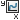(MotionManager toolbar).
2. In the PropertyManager under Result, for Category, select Displacement/Velocity/Acceleration.
3. For Subcategory, select one of the following:
• Linear Displacement
• Linear Velocity
• Linear Acceleration
4. For Select result component, select an option:
• X Component, Y Component, or Z Component to calculate the magnitude of the result in the selected direction.
Optionally, you can select a reference partto plot the result relative to the coordinates of that part.
• Magnitude to resolve the magnitude in the direction of the result vector in rectangular coordinates.
• Radial Component, (Linear Velocity only), to resolve the radial component of the velocity vector in polar coordinates.
• Tangential Component, (Linear Acceleration only), to specify the component of the acceleration vector tangent to the path of motion.
• Normal Component, (Linear Acceleration only), to specify the component of the acceleration vector normal to the path of motion.
5. Select features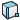:
• Select one part face or point or vertex, to calculate the result for motion of the part with respect to the assembly origin.
• Select two part faces or points or vertices, to calculate the result for the motion of the origin of the first selected part with respect to that of the origin of the second selected part.
• Select a motor to calculate the result for the motion of the location point for the motor with respect to its initial position. You define the motor location point when you specify the motor in the Motor PropertyManager. You can optionally define the frame of reference for the motor motion in the Motor PropertyManager by selecting a component for Component to Move Relative to, or define the frame of reference from the assembly origin by leaving Component to Move Relative to blank.
You can also select a force or other motion element to calculate the result for the point of application of the motion element.
You can suppress the motion element and still calculate the result for the point of application of the motion element.
• Select a mate to calculate the result of the relative motion of the geometric center of the first entity defining the mate with respect to the geometric center of the second entity defining the mate. You specify the mated entities when you define the mate in the Mate PropertyManager. The result is computed in the local direction of the mate unless you select X Component, Y Component, or Z Component for the result component.
The geometric center of an axis is at the center of the axis.
Selected part origins are indicated with a small ball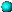.
6. Select the Plot Results options you require, and click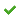.
The result appears in the MotionManager tree.
7. Click Calculate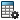(MotionManager toolbar).